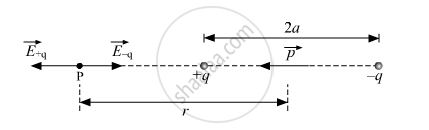Share

# An Electric Dipole of Dipole Moment P Consists of Point Charges +Q and −Q Separated by a Distance 2a Apart. Deduce the Expression for the Electric Field E Due to the Dipole at a Distance X from the Centre of the Dipole on Its Axial Line in Terms of the Dipole Moment P - CBSE (Science) Class 12 - Physics

#### Question

An electric dipole of dipole momentvecp consists of point charges +q and −q separated by a distance 2a apart. Deduce the expression for the electric field vecE due to the dipole at a distance x from the centre of the dipole on its axial line in terms of the dipole moment vecp. Hence show that in the limit x>> a, vecE->2vecp"/"(4piepsilon_0x^3)

#### Solution

Electric Field on Axial Line of an Electric DipoleLet P be at distance r from the centre of the dipole on the side of charge q. Then, the electric field at point P due to charge q of the dipole is given by

vecE_-q=-q/(4piepsilon_0(r+a)^2)hatp

Where hatp is the unit vector along the dipole axis (from − q to q).

Also, the electric field at point P due to charge +q of the dipole is given by

vecE_+q=q/(4piepsilon_0(r-a)^2)hatp

The total field at P is

vecE=vecE_+q+vecE_-q=q/(4piepsilon_0)[1/(r-a)^2-1/(r+a)^2]hatp

=>vecE=q/(4piepsilon_0)(4ar)/(r^2-a^2)^2hatp

Given:

r = x

vecE=q/(4piepsilon_0)(4ax)/(x^2-a^2)^2hatp

For x >> a,

vecE=(4qa)/(4piepsilon_0x^3)hatp

vecE=(2vecp)/(4piepsilon_0x^3)" "[.:vecp=(qxx2a)hatp]

Is there an error in this question or solution?

#### Video TutorialsVIEW ALL 

Solution An Electric Dipole of Dipole Moment P Consists of Point Charges +Q and −Q Separated by a Distance 2a Apart. Deduce the Expression for the Electric Field E Due to the Dipole at a Distance X from the Centre of the Dipole on Its Axial Line in Terms of the Dipole Moment P Concept: Electric Dipole.
S